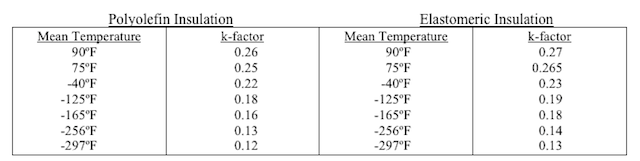# Insulation Ratings: R Factor, K Factor & C FactorInsulation terms can be quite confusing to anyone outside the industry. If you’ve ever bought insulation for your house, you know that insulation with a high R factor is better. But what, exactly, does that mean? Did you know that the R Factor depends on other factors?

When it comes to buying more specific insulation products, like removable insulation jackets for hot pipes, understanding the particulars of the three measures of insulation is key. In order to understand the well-known R factor it’s important to understand the factors upon which it relies, the K factor and C factor.

If you are seeking out the formulas to calculate these factors, check out our R, C & K Factor Formula Conversion Table that lists all the formulas discussed in this article. For more information, read on!

I Want
K FactorC FactorR Factor
I HaveK FactorC=K-factor/in. of thicknessR= in. of thickness/K-factor
C FactorK=C-factor in. of thicknessR=1/C-factor
R FactorK=in. of thickness / R-factorC-1/R-factor
None of
the Above
K=BTU-in / hr – ft² – °FC=BTU/(hr · ft · °F)R=h · ft² · °F/BTU

### The K Factor of Insulation

#### What is the K Factor of Insulation?

The K factor of insulation represents the material’s thermal conductivity or ability to conduct heat. Usually, insulation materials have a K Factor of less than one.  The lower the K factor, the better the insulation. The textbook definition of the K factor is “The time rate of steady heat flow through a unit area of homogeneous material induced by a unit temperature gradient in a direction perpendicular to that unit area.”  That’s a mouthful.

Simplified, the K factor is the measure of heat that passes through one square foot of material that is one inch thick in an hour.

#### How Do I Calculate the K Factor of Insulation?

If R factor is unknown, the formula to calculate the K factor of insulation is:

K factor = BTU-in / hr – ft– °F
or
British Thermal Unit-Inch Per Square Foot Per Hour Per Fahrenheit Degree

If R factor is know, this easier formula can be used to calculate the K factor:

K factor = inches of thickness / R Factor

#### How is the K Factor of Insulation Reported?

K factors are reported at one or many mean temperatures. The mean temperature is the average of the sum of the hottest and coldest surface temperatures which the insulation material is exposed to.

Put more simply, the testing apparatus that determines the K factor of an insulation material places a sample of the material between two plates, hot & cold, and the average of the surface temperatures of those two plates equals the mean temperature. Here is an example of an insulation material’s K factor report:via Nomaco Insulation

Notice that as the mean temperature rises, so does the K factor. It’s important to observe the K factor & mean temperature when comparing insulation.

### The C Factor of Insulation

#### What is the C Factor of Insulation?

The C factor stands for Thermal Conductance Factor. The C factor, like the K factor, is a rate of heat transfer through a material. The lower the C factor, the better the insulating properties of the material. It is the quantity of heat that passes through a foot of insulation material.

The C factor is dependent upon the thickness of the insulation. The thicker the insulation is, the lower the C factor will be and thus the better the material will be at insulating. This is one of the main differences between the K factor and C factor, because generally the thickness of an insulation material will not affect its K factor.

#### How Do I Calculate the C Factor of Insulation?

If the K factor is unknown, the formula to calculate the C factor of insulation is:

BTU/(hr·ft⋅°F)
or
Btus/hour per square foot per degree F of temperature difference

If the K factor is known, this easier formula can be used:

C factor = K factor / inches of thickness

### The R Factor

#### What is the R Factor of Insulation?

The R factor pulls together all of the information of the other factors and makes it easy to judge the effectiveness of insulating material. The R factor of insulation can be found most easily of the insulation factors discussed, and it is the most popular indicator of a material’s insulator properties. Generally it is listed on an insulation material’s label. The R factor stands for thermal resistance. The higher the R factor, the better the insulation.

The textbook definition for R Factor is: the quantity determined by the temperature difference, at steady state, between two defined surfaces of a material or construction that induces a unit heat flow through a unit area. Aren’t textbooks supposed to be helpful?

To simplify, the R factor is a variable value that measures the ability of a material to block heat rather than radiate it. The variable is the C factor, which is dependent upon the thickness of the material. It is the opposition to the flow of heat energy.

#### How Do I Calculate the R Factor of Insulation?

There are a few formulas to calculate the R factor of insulation, depending on if your K factor and C factor are known. If they are unknown, you can use this formula:

h·ft²·°F/Btu
or
degrees F times square feet of area times hours of time per Btus of heat flow

If your K factor and C factor are known, you can use these formulas which may be easier to use:

R-factor = 1 / C-factor
or
R-factor = thickness in inches / K-factor

Keep in mind that these factors are specific to the materials being measured. Understanding the ins and outs of the factors that help describe how effective insulation material is will go a long way to helping make the buying process easier.

This entry was posted in Recent News & Updates. Bookmark the permalink.